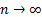/

### Extreme Events – Specimen Question A.2.1

You are an investor seeking to understand the behaviour of Index A:

(a)    Calculate the mean, (sample) standard deviation, skew and (excess) kurtosis of its log returns over the period covered by the above table.

(b)   Do the statistics calculated in (a) appear to characterise a fat-tailed distribution if we adopt the null hypothesis that the log returns would otherwise be coming from a normal distribution and we use the limiting form of the distributions for these test statistics (i.e. the form ruling when,  whereis the number of observations)?

(c)    Prepare a standardised quantile-quantile plot for Index A. Does it appear to be fat-tailed?

(d)   Does the Cornish-Fisher 4th moment approximation appear to under or overstate the fat-tailed behaviour of this series?

(e)   What other methodologies could you use to formulate a view about how fat-tailed this return series might be if your focus was principally on fat-tailed behaviour around or below the lower 10th percentile quantile level?